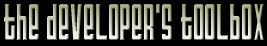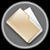This section of the archives stores flipcode's complete Developer Toolbox collection, featuring a variety of mini-articles and source code contributions from our readers.1-D Generalized Wavelet Class   Submitted byThis is just some basic 1-d Wavelet transform code. Pretty simple and effective, but it leaves a lot of figuring out to the programmer. I don't include anything but the transform code encapsulated in a class so that you can classify a wavelet rather than the data it operates on. Good for adaptive methods and best-basis searches and so on. Fortunately, there are ways to deal with that. I personally plugged this code into a simple Java applet that performs the transform on some pre-defined function-produced data, and make sure it all works out. If not, I tweak offset parameters and so on. I also set it up to save a limited number of coefficients (tuned by a slider bar) so that I can actually visualize the wavelets themselves. Some can look weird. Filters coefficients themselves, you can get from various places like the Bath Wavelet Warehouse and so on. By default, this code goes to a 2nd-order Daubechies wavelet (4 taps). But here are a few others you can try --
 ```Haar (0th order) (Identical to the sum+difference transform) H = {1/SQRT_2, 1/SQRT_2} Ht = H all offsets = 0 imsG = 1 imsGt = 1Daubechies-6 (3rd order) H = {0.33267055295008324, 0.8068915093110934, 0.4598775021184894, -0.13501102001025506,-0.08544127388202508, 0.0352262918557091}; Ht = H OffH = 1 OffG = 3 OffHt = 1 OffGt = 3 imsG = 1 imsGt = 1Daubechies-8 (4th order) H = {0.2303778133088964, 0.7148465705529154, 0.6308807679398587, -0.0279837694168599, -0.1870348117190931, 0.0308413818355607, 0.0328830116668852, -0.0105974017850690} Ht = H OffH = 1 OffG = 5 OffHt = 1 OffGt = 5 imsG = 1 imsGt = 1B-Spline_3_3 H = {SQRT2 * 3.0 / 64.0, SQRT2 * -9.0 / 64.0, SQRT2 * -7.0 / 64.0, SQRT2 * 45.0 / 64.0, SQRT2 * 45.0 / 64.0, SQRT2 * -7.0 / 64.0, SQRT2 * -9.0 / 64.0, SQRT2 * 3.0 / 64.0} Ht = {SQRT2 * 1.0 / 8.0, SQRT2 * 3.0 / 8.0, SQRT2 * 3.0 / 8.0, SQRT2 * 1.0 / 8.0} OffH = 3 OffHt = 1 OffG = 1 OffGt = 3 imsG = 1 imsGt = 1B-Spline_3_7 (This one has a very convenient imperfection that seems to cause a weird but very mild boost in audio fidelity when you do nothing but transform and inverse transform ^__^) H ={ SQRT2 * -35.0 / 16384, SQRT2 * -105.0 / 16384, SQRT2 * -195.0 / 16384, SQRT2 * 865.0 / 16384, SQRT2 * 363.0 / 16384, SQRT2 * -3489.0 / 16384, SQRT2 * -307.0 / 16384, SQRT2 * 11025.0 / 16384, SQRT2 * 11025.0 / 16384, SQRT2 * -307.0 / 16384, SQRT2 * -3489.0 / 16384, SQRT2 * 363.0 / 16384, SQRT2 * 865.0 / 16384, SQRT2 * -195.0 / 16384, SQRT2 * -105.0 / 16384, SQRT2 * -35.0 / 16384} Ht = same as Ht for Spline_3_3 OffH = 7 OffHt = 1 OffG = 1 OffGt = 7 imsG = 1 imsGt = 1 ```

There's a funny bit of Pascal's Triangle action going on in all of 'em. Albeit hard to see in the Daubechies cases since I'm just giving you straight values. Anyway, it's a nice little thing to study with, and it's fast. When I tried a lazy compressor, the profiling showed that the greatest amount of time was being eaten up by a selection sort routine. Anyway, good luck in your research if you use this. *waves*

Currently browsing [WaveElement.zip] (1,931 bytes) - [WaveElement.h] - (1,616 bytes)

 ```// WaveElement.h: interface for the WaveElement class. // ////////////////////////////////////////////////////////////////////// #ifndef WaveElement_H #define WaveElement_H#ifndef WaveConstants #define WaveConstants const float SQRT2 = (float)1.41421356237309504880168; // might be useful const float SQRT3 = (float)1.73205080756887729352745; // for basis defining const float fpi = (float)3.1415926535897932384626433; const short PktSize = 256; // Size of packets in # of samples #endif#define DllExport __declspec( dllexport ) // Define a macro for exporting to dll class DllExport WaveElement { public: WaveElement(); // By default, it's Daubechies-4 wavelet. (2nd-order) WaveElement(char str[], float f1[], float f2[], int o1, int o2, int o3, int o4, int s1, int s2); WaveElement(char str[], float f1[], float f2[], int o1, int o2, int o3, int o4, int s1, int s2, short l1, short l2); int Mod(int n, int m); void Decompose(float inp[], float out[]); // Wavelet transform void Compose(float inp[], float out[]); // Inverse transform char Name; // Filter name -- just to show on the screen. float Hfilt; // I'm assuming no farther than 10th order float Htfilt; // which at the most has 24 coefficients... // depending on family, anyway. int offH, offG, offHt, offGt; // offsets to look through for the transforms int imsG, imsGt; // initial sign convention short lenH, lenHt; // actual used number of coefficients. };#endif ```

Currently browsing [WaveElement.zip] (1,931 bytes) - [WaveElement.cpp] - (3,307 bytes)

 ```// WaveElement.cpp: implementation of the WaveElement class. // ////////////////////////////////////////////////////////////////////// #include "WaveElement.h" #include #include WaveElement::WaveElement() { int i; strcpy(Name,"Daubechies-4"); for(i = 0; i < 25; i++) { Hfilt[i] = (float)0.0; Htfilt[i] = (float)0.0; } Hfilt = (float)SQRT2 * (1 + SQRT3) / 8; Htfilt = Hfilt; Hfilt = (float)SQRT2 * (3 + SQRT3) / 8; Htfilt = Hfilt; Hfilt = (float)SQRT2 * (3 - SQRT3) / 8; Htfilt = Hfilt; Hfilt = (float)SQRT2 * (1 - SQRT3) / 8; Htfilt = Hfilt; offH = 1; offG = 1; offHt = 1; offGt = 1; imsG = 1; imsGt = 1; lenH = 4; lenHt = 4; }WaveElement::WaveElement(char str[], float f1[], float f2[], int o1, int o2, int o3, int o4, int s1, int s2) { int i; strcpy(Name,str); lenH = sizeof(f1) / sizeof(float); lenHt = sizeof(f2) / sizeof(float); for(i = 0; i < lenH; i++) Hfilt[i] = f1[i]; for(i = 0; i < lenHt; i++) Htfilt[i] = f2[i]; offH = o1; offG = o2; offHt = o3; offGt = o4; imsG = s1; imsGt = s2; }WaveElement::WaveElement(char str[], float f1[], float f2[], int o1, int o2, int o3, int o4, int s1, int s2, short l1, short l2) { int i; strcpy(Name, str); lenH = l1; lenHt = l1; for(i = 0; i < lenH; i++) Hfilt[i] = f1[i]; for(i = 0; i < lenHt; i++) Htfilt[i] = f2[i]; offH = o1; offG = o2; offHt = o3; offGt = o4; imsG = s1; imsGt = s2; }int WaveElement::Mod(int n, int m) { n = n % m; if(n < 0) n += m; return n; }void WaveElement::Decompose(float inp[], float out[]) { int i, j, pos; int res, lh, lg, sg; float sum; float tmp[PktSize]; res = PktSize; lh = lenH; lg = lenHt; for(i = res-1; i>= 0; i--) { out[i] = inp[i]; // initial copying tmp[i] = (float)0.0; // get rid of garbage } for(res /= 2; res > 0; res /= 2) { for(i = 0; i < res; i++) { // H filter sum = (float)0.0; for(j = 0; j < lh; j++) { pos = Mod(i+i+j - offH, res+res); sum += Hfilt[j] * out[pos]; } tmp[i] = sum; // G filter sum = (float)0.0; sg = imsG; for(j=0;j=0; i--) out[i] = tmp[i]; } }void WaveElement::Compose(float inp[], float out[]) { int i,j; int res, resmax, pos, lht, lgt, sgt; float tmp[PktSize]; float val; resmax = PktSize >> 1; lht = lenHt; lgt = lenH; for(i = 0; i < PktSize; i++) tmp[i] = (float)0.0; out = inp; for(res = 1; res <= resmax; res += res) { for(i = 0; i < res; i++) { // H~ filter val = out[i]; for(j = 0; j < lht; j++) { pos = Mod(i+i+j - offHt, res+res); tmp[pos] += Htfilt[j] * val; } // G~ filter sgt = imsGt; val = inp[i+res]; for(j = 0; j < lgt; j++) { pos = Mod(i+i+j - offGt, res+res); tmp[pos] += sgt * Hfilt[lgt-1-j] * val; sgt = -sgt; } } for(i = res+res-1; i >= 0; i--) { out[i] = tmp[i]; tmp[i] = (float)0.0; } } } ```

The zip file viewer built into the Developer Toolbox made use of the zlib library, as well as the zlibdll source additions.